R -4- Dataframes

Dataframes are a central type of object when working with statistics in R. They are actually simple tables or two-dimensional matrices arrays which contain your data, arranged in a rather specific manner. Here we’ll see what they are made of, how to handle them and how to make the best of it to analyse your precious data.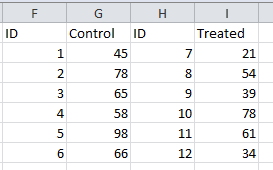A dataframe looks like a two-dimensional matrix. However, unlike a matrix, a dataframe can contain more than numerical values. You can also fill in columns with text values and boolean/logical values (TRUE and FALSE). In a dataframe, the variables, dependent and independent, are placed in columns, while the rows represent the […]

1. Structure and technical properties of a dataframe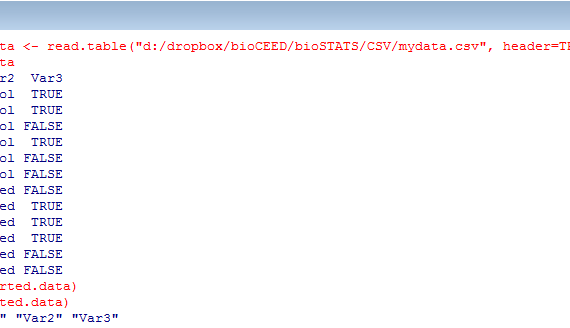Most of the time you will load the dataframe from an external file, most likely a text file, an excel file or a CSV file (Comma-Separated Values). In that case, you may follow this procedure. In brief, you have to use the command read.xls(), read.table() or read.csv() to open a file and transfer its […]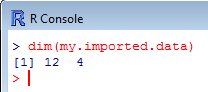From here, you can use summary() to get a quick overview of your imported dataframe. summary() offers a reduced, but in fact very informative list of descriptive statistics for each numerical variable, including mean, median, quartiles and minimum/maximum. As for the text and logical variables, a count of each level […]

3. Summary of the dataframe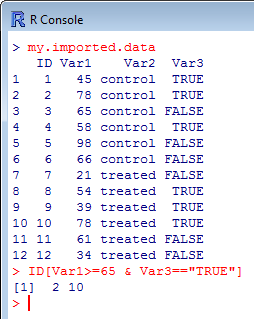R gives you the possibility to extract parts of your dataframe. This can be useful to isolate data elements, columns of variables, subsets in your sample… Here are a few commands that create subsets from the dataframe.   extracting single data elements Type the name of the object followed by […]

4. Subscripts of a dataframe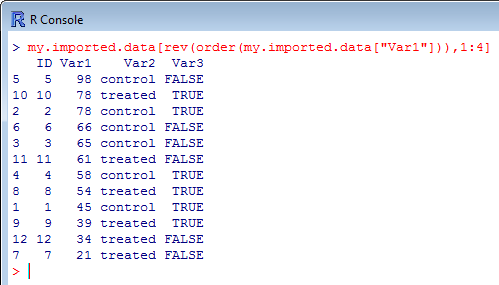It is not because you have imported your data from an external source that the order of the observations has to stay as it originally was. You can sort the observations based on ascending or descending values in a column. To do so, you will use the function object.name[order(object.name[,X]),a:b] where X […]

5. Sorting data in the dataframe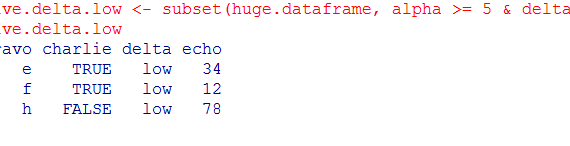When working with big (or huge) dataframes, it might be convenient to create a new dataframe that contains parts of the original one which are selected based on variable names, specific columns or rows, values or ranges… In this post, we will use the following “pseudo-huge” dataframe as an example: […]

6. Working on a subset of the dataframe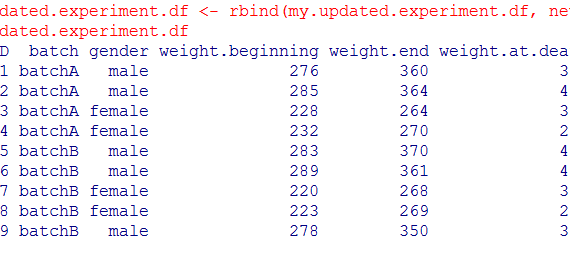It is fairly easy to build a dataframe from scratch in R. All you need is a series of vectors and/or series containing your data and a good recipe! And very often, a good recipe is a simple one… First you have to decide how many columns this dataframe will have, […]